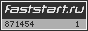# ФОТОКНИГА

## Книги по фотографии и фотоальбомы.Профессионалам и любителям

Фотография: литература книги учебники пособия справочники по фотографии / цифровая фотография / профессиональная фотография / любительская фотография / подарочные фотоальбомы / старые и старинные фотографии# Mathematical Models in Photographic Sciences (Mathematics in Industry)Искать в интернет-магазинах Страниц: 178Book DescriptionThis book presents mathematical models that arise in current photographic science. The book contains seventeen chapters, each dealing with one area of photographic science, and a final chapter containing exercises. Each chapter, except the two introductory chapters and the last one, begins with general background information at a level understandable by graduate and undergraduate students. It then proceeds to develop a mathematical model, using mathematical tools such as ordinary differential equations, partial differential equations, and stochastic processes. Next, some mathematical results are mentioned, often providing a partial solution to problems raised by the model. Finally, most chapters include open problems. The last chapter of the book contains "Modeling and Applied Mathematics" exercises based on the material presented in the earlier chapters.These exercises are intended primarily for graduate students and advanced undergraduates. Категории каталога:Оборудование; Свет и проявка

 [ Бестселлеры ]

Фотокалейдоскоп
Про инфракрасную фотографию глазами дилетанта

Про инфракрасную фотографию глазами дилетанта

> info@bibliophoto.ru <